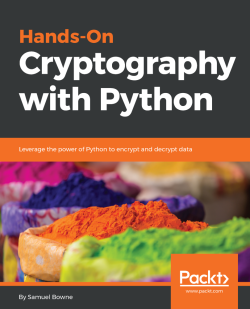### Hands-On Cryptography with Python4.5 (2 reviews total)
By Sam Bowne

• Constantly updated with 100+ new titles each month
• Breadth and depth in over 1,000+ technologies

Cryptography is essential for protecting sensitive information, but it is often performed inadequately or incorrectly.

Hands-On Cryptography with Python starts by showing you how to encrypt and evaluate your data. The book will then walk you through various data encryption methods,such as obfuscation, hashing, and strong encryption, and will show how you can attack cryptographic systems. You will learn how to create hashes, crack them, and will understand why they are so different from each other. In the concluding chapters, you will use three NIST-recommended systems: the Advanced Encryption Standard (AES), the Secure Hash Algorithm (SHA), and the Rivest-Shamir-Adleman (RSA).

By the end of this book, you will be able to deal with common errors in encryption.

Publication date:
June 2018
Publisher
Packt
Pages
100
ISBN
9781789534443

## Chapter 1. Obfuscation

Python is the best language to start with if you are a beginner, which is what makes it so popular. You can write powerful code with just a few lines, and most importantly, you can handle arbitrarily large integers with complete precision. This book covers essential cryptography concepts; classic encryption methods, such as the Caesar cipher and XOR; the concepts of confusion and diffusion, which determine how strong a crypto system is; hiding data with obfuscation; hashing data for integrity and passwords; and strong encryption methods and attacks against these methods, including the padding oracle attack. You do not need to have programming experience to learn any of this. You don't need any special computer; any computer that can run Python can do these projects. We'll not be inventing new encryption techniques just for learning how to use standard pre-existing ones that don't require anything more than very basic algebra.

We will first deal with obfuscation, the basic idea of what encryption is, and old-fashioned encryption techniques that hide data to make it more difficult to read. This latter process is one of the basic activities that encryption modules use in combination with other methods to make stronger, more modern encryption techniques.

In this chapter, we will cover the following topics:

• Installing and setting up Python
• Caesar cipher and ROT13
• base64 encoding
• XOR

The term crypto has become overloaded recently with the introduction of all currencies, such as Bitcoin, Ethereum, and Litecoin. When we refer to crypto as a form of protection, we are referring to the concept of cryptography applied to communication links, storage devices, software, and messages used in a system. Cryptography has a long and important history in protecting critical systems and sensitive information.

During World War II, the Germans used Enigma machines to encrypt communications, and the Allies went to great lengths to crack the encryption. Enigma machines used a series of rotors that transformed plaintext to ciphertext, and by understanding the position of the rotors, the Allies were able to decrypt the ciphertext into plaintext. This was a momentous achievement but took significant manpower and resources. Today it is still possible to crack certain encryption techniques; however, it is often more feasible to attack other aspects of cryptographic systems, such as the protocols, the integration points, or even the libraries used to implement cryptography.

Cryptography has a rich history; however, nowadays, you will come across new concepts, such as blockchain, that can be used as a tool to help secure the IoT. Blockchain is based on a set of well-known cryptographic primitives. Other new directions in cryptography include quantum-resistant algorithms, which hold up against a theorized onslaught of quantum computers and quantum key distributions. They use protocols such as BB84 and BB92 to leverage the concepts of quantum entanglement and create good-quality keys for using classical encryption algorithms.

## Installing and setting up Python

Python has never been easy to install. In order to proceed, let's make sure that we have set up Python on our machine. We will see how to use Python on macOS or Linux and how to install it on Windows.

### Using Python on Mac or Linux

On a macOS or Linux system, you do not need to install Python because it is already included. You just need to open a Terminal window and enter the `python` command. This will put you in an interactive mode where you can execute `python` commands one by one. You can close the interactive mode by executing the `exit()` command. So, basically, to create a script, we use the`nano`text editor followed by the name of the file. We then enter `python` commands and save the file. You can then run the script with`python`followed by the script name. So, let's see how to use Python on macOS or Linux in the following steps:

1. Open the Terminal on a macOS or Linux system and run the `python` command. This opens an interactive mode of Python, as shown in the following screenshot: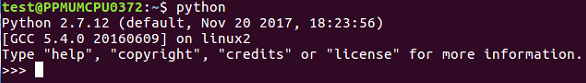1. When you use the `print` command, it prints `Hello` right away:
```>>> print "Hello"
Hello```
1. We will then leave with the following command:
`>>> exit()`
1. As mentioned before, to use Python in interactive mode, we will enter the command as shown:
`\$ nano hello.py`
1. In the `hello.py` file, we can write commands like this:
`print "HELLO"`
1. Save the file by pressing Ctrl + X followed by Y and Enter only if you've modified it.
2. Now, let's type Python followed by the the script name:
`\$ python hello.py`

When you run it, you will get the following output: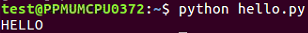The preceding command runs the script and prints out `HELLO`; that's all you have to do if you have a macOS or Linux system.

### Installing Python on Windows

Here are the steps which you need to follow:

2. Run it in a Command Prompt window
3. Start interactive mode with Python
4. Close with `exit()`

To create a script, you just use Notepad, enter the text, save the file with Ctrl + S, and then run it with `python` followed by the script name. Let's get started with the installation.

Open the Python page using link given previously and download Python. It offers you various versions of Python. In this book, we will use Python 2.7.12.

Sometimes, you can't install it right away because Windows marks it as untrusted:

1. You have to unblock it in the properties first so that it will run, and run the installer
2. If you go through the steps of the installer, you'll see an optional step named `Add python.exe to path`. You need to choose that selection

The purpose of that selection is to make it so Python can run from the command line in a Terminal window, which is called Command Prompt on Windows.

Now let's proceed with our installation:

1. Open the Terminal and type the following command:
`\$ python`
1. When you run it, you can see that it works. So, now we will type a command:
`print "HELLO"`

Refer to the following screenshot: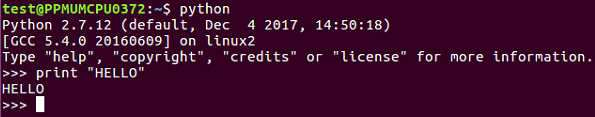1. We can exit using the `exit()` command as shown earlier.
2. Now, if we want to make a script, we type the following command:
`notepad hello.py`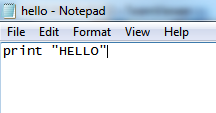1. We want to create a file. In that file, we enter the following command:
`print "HELLO"`
1. Then, save and close it. In order to run it, we need to enter the following command:
`\$ python hello.py`

It runs and prints `HELLO`.

Usually, when you install Python on Windows, it fails to correct the path, so you have to execute the following commands to create a symbolic link; otherwise, Python will not start correctly from the command line:

1. `cd c: \Windows`
2. `mklink /H python.exe`
3. `c: \python27\python.exe`

In the next section, we will look at the Caesar cipher and ROT13 obfuscation techniques.

## Caesar cipher and ROT13

In this section, we will explain what a Caesar cipher is and how to implement it in Python. Then, we will consider other `shift` values, modular arithmetic, and ROT13.

A Caesar cipher is an ancient trick where you just move every letter forward three characters in the alphabet. Here is an example:

• Plaintext: `ABCDEFGHIJKLMNOPQRSTUVWXYZ`
• Ciphertext: `DEFGHIJKLMNOPQRSTUVWXYZABC`

So, `HELLO` becomes `KHOOR`.

To implement it, we're going to use the `string.find()` method. The interactive mode of Python is good for testing new methods, hence it's easy to create a string. You can make a very simple script to implement the Caesar cipher with a string named`alpha`for alphabet. You can then take input from the user, which is the plaintext method, then set a value,`n`, which equals the length of the string, and the string out is equal to an empty string. We then have a loop that goes through`n`repetitions, finding the character from string in and then finding the location of that character in the `alpha` string. It then prints out those three values so that we can make sure that the script is working correctly, then it adds`3`to `loc`(location) and puts the corresponding character in string out, and again prints out partial values so that we can see that the script is working correctly. At the end, we print our final output. Adding extra print statements is a very good way to begin your programming because you can detect mistakes.

### Implementing the Caesar cipher in Python

Let's go ahead and open the Terminal and follow these steps to implement Caesar cipher in Python:

1. We will use Python in interactive mode first and then make a string that just has some letters in order to test this method:
```>>> str = "ABCDE"
>>> str.find("A")
0
>>> str.find("B")
1
>>> exit()```
1. Because we understand how the string methods work, we'll exit and go into the `nano` text editor to look at the first version of our script:
`\$ nano caesar1.py`
1. When you run the command, you will get the following code:
```alpha = "ABCDEFGHIJKLMNOPQRSTUVWXYZ"
str_in = raw_input("Enter message, like HELLO: ")

n = len(str_in)
str_out = ""

for i in range(n):
c = str_in[i]
loc = alpha.find(c)
print i, c, loc,
newloc = loc + 3
str_out += alpha[newloc]
print newloc, str_out

print "Obfuscated version:", str_out```

You can see the alphabet and the input from the user in the script. You calculate the length of the string, and for each character, `C` is going to be the one character on processing, `loc` will be the numerical location of that character, `newloc` will be `loc` plus `3`, and we can then add that character to string out. Let's see this.

1. Leave using Ctrl+X and then enter the following command:
`\$ python caesar1.py`
1. When you run this command, you will get the following output:
`Enter message, like HELLO:`
1. If we enter `HELLO`, it prints out the correct answer of `KHOOR`: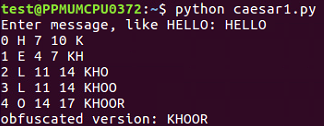When we run this script, it takes the input of `HELLO` and it breaks it up character by character so that it processes each character on a separate line. `H` is found to be the 7th character, so adding `3` gives me `10`, which results in `K`. It shows us character by character how it works. So, the first version of the script is a success.

To clean the code further, we will remove the unnecessary `print` statements and switch to a `shift` variable. We will create a variable `shift` variable. Which also comes from raw inputs, but we have to convert it to an integer because raw input is interpreted as `text` as you can't add `text` to an integer. This is the only change in the script that follows. If you give it a`shift`value of`3`, you get`KHOOR`; if you give it a`shift`value of`10`, you get`ROVVY`; but if you put in a`shift`value of`14`, it crashes, saying string index out of range. Here, the problem is, we've added multiple times to the`loc` variable, and eventually, we move past`Z`, and the variable is no longer valid. In order to improve that, after adding something to the variable, we'll check to see whether it's greater than or equal to`26`, and whether `26` can be subtracted from it. Once you run this, you can use a shift of`14`, which will work. We can use a shift of`24`, and it works too. However, if we use a shift of`44`, it's out of range again. This is because just subtracting`26`once when it's over`26`is not really enough, and the right solution here is modular arithmetic. If we put`% 26`, it will calculate the number modulus`26`, which will prevent it from ever leaving the range of`0`through`25`. It will divide it by`26`and keep only the remainder, as expected in this case. We're going to see the modular function many more times as we move forward in cryptography. You can put in any`shift` value of your choice, such as `300`, and it will never crash, but will turn that into a number between`0`and`25`.

Let's see how the script works with other shift values:

1. Take a look at the script Caesar:
`\$ nano caesar2.py`
1. When you run it, you will get the following: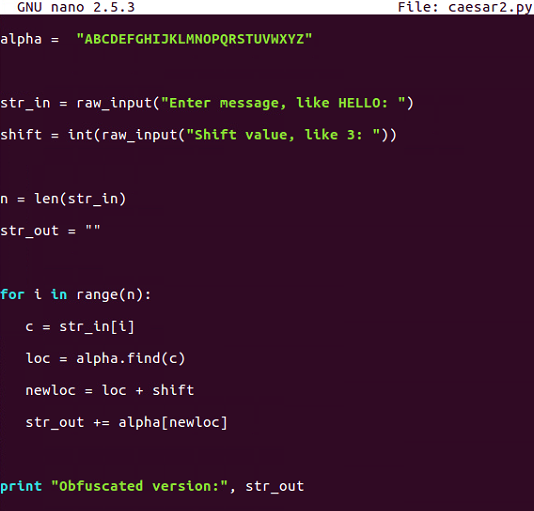1. This is the script that allows us to vary the `shift` value but does not handle anything about the `shift` value getting too large. Let's run the following command:
`\$ python caesar2.py`
1. If you enter `HELLO` and give it a shift of `3`, it's fine, but if we run it again and give it a shift of `20`, it crashes: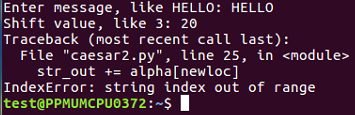So, as expected, there are some limitations in this one.

1. Let's move on to `caesar3`:
`\$ nano caesar3.py`
1. After running it, we get the following output: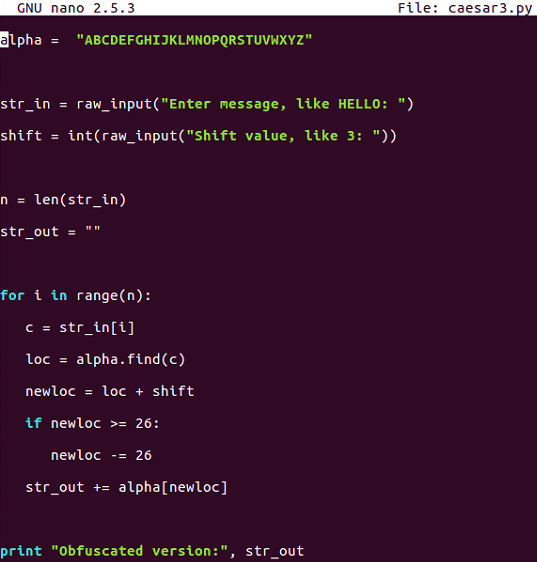`Caesar3` attempts to solve that problem by catching it if we know that the addition causes it to be greater than or equal to `26` and subtracting `26` from it.

1. Let's run the following command:
`\$ python caesar3.py`
1. We will give it `shift` characters and a `shift` of `20`, and it will be fine: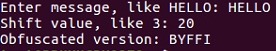1. If we give it a shift of `40`, it does not work: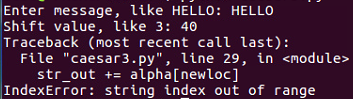There is some improvement, but we are still not able to handle any value of `shift`.

1. Let's go up to `caesar4`:
`\$ nano caesar4.py`
1. When you run the command, you will get this: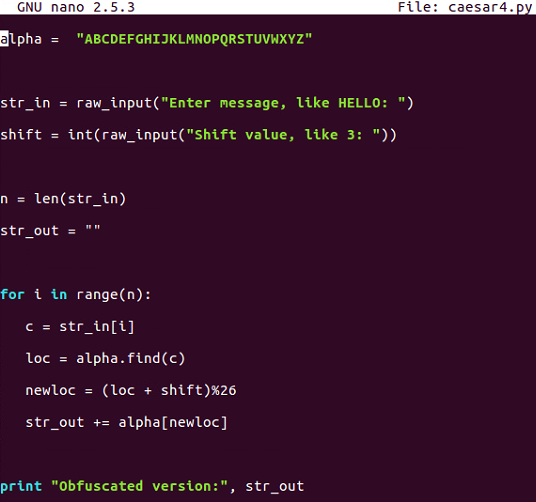This is the one that uses modular arithmetic with the percent sign, and that's not going to fail.

1. Let's run the following command:
`\$ python caesar4.py`
1. When you run the command, you will get this: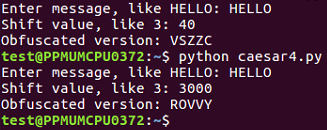This is the script that handles all the values of the Caesar shift.

### ROT13

ROT13 is nothing more than a Caesar cipher with a `shift` equal to `13` characters. In the script that follows, we will hardcode the shift to be`13`. If you run one cycle of ROT13, it changes`HELLO`to`URYYB`, and if you encrypt it again with the same process, putting in that`URYYB`, it'll turn back into`HELLO`, because the first shift is just by`13`characters and shifting by another`13`characters takes the total shift to`26`, which wraps right around, and that is what makes this one useful and important:

1. Now let's look at the ROT13 script using the following command:
`\$ nano rot13.py`
1. When you run the preceding command, you can see the script file: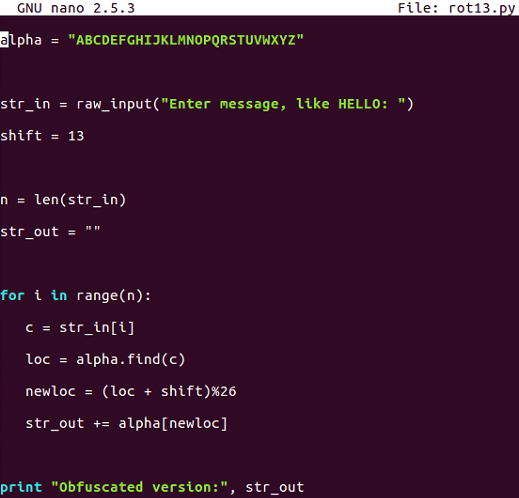1. It's just exactly equal to our last Caesar cipher shift, with a script with a shift of `13`. Run the script as shown here:
`\$ python rot13.py`

The following is the output: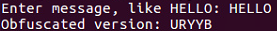1. If we enter the message `URYYB` and run that, it turns back into `HELLO`: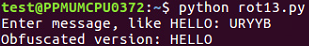This is important because there are quite a few cryptographic functions that have this property; where you encrypt something once and encrypt it again, you reverse the process. Instead of making it more encrypted, it becomes unencrypted. In the next section, we will cover base64 encoding.

## base64 encoding

We will now discuss encoding ASCII data as bytes and base64 encoding these bytes. We will also cover base64 encoding for binary data and decoding to get back to the original input.

### ASCII data

In ASCII, each character turns into one byte:

• `A` is `65` in base `10`, and in binary, it is `0b01000001`. Here, you have `0` in the most significant bit because there's no `128`, then you have `1` in the next bit for `64` and `1` in the end, so you have 64 + 1=65.
• The next is `B` with base `66` and `C` with base `67`. The binary for `B` is `0b01000010`, and for `C`, it is `0b01000011`.

The three-letter string `ABC` can be interpreted as a 24-bit string that looks like this: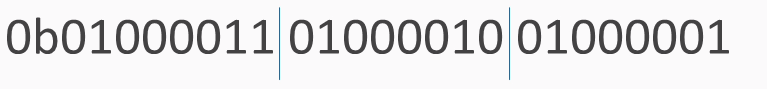We've added these blue lines just to show where the bytes are broken out. To interpret that as base64, you need to break it into groups of 6 bits. 6 bits have a total of 64 combinations, so you need 64 characters to encode it.

The characters used are as follows: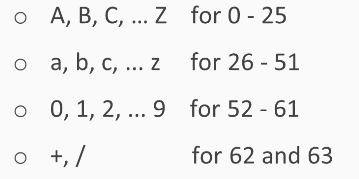We use the capital letters for the first 26, lowercase letters for another 26, the digits for another 10, which gets you up to 62 characters. In the most common form of base64, you use `+` and `/` for the last two characters: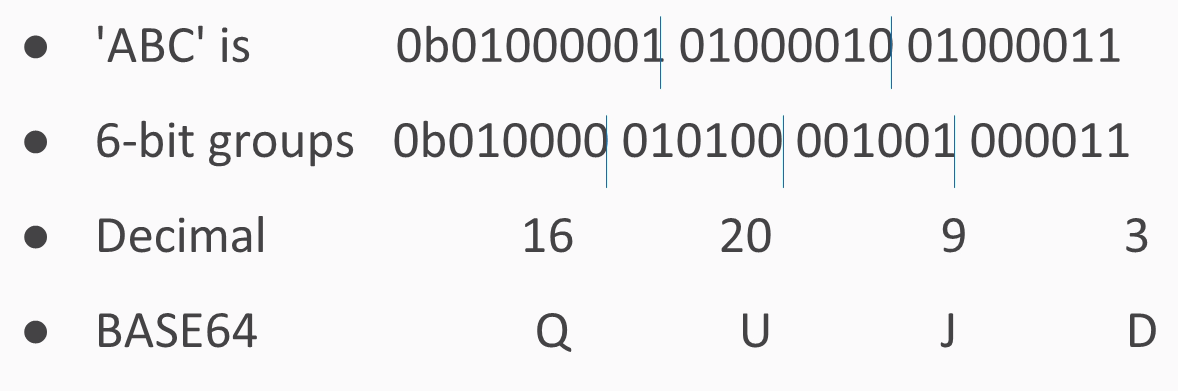If you have an ASCII string of three characters, it turns into 24 bits interpreted as 3 groups of 8. If you just break them up into 4 groups of 6, you have 4 numbers between 0 and 63, and in this case, they turn into `Q``U``J`, and `D`. In Python, you just have a string followed by the command:

```>>> "ABC".encode("base64")
'QUJD\n'```

This will do the encoding. Then add an extra carriage return at the end, which neither matters nor affects the decoding.

What if you have something other than a group of 3 bytes?

### Note

The `=` sign is used to indicate padding if the input string length is not a multiple of 3 bytes.

If you have four bytes for the input, then the base64 encoding ends with two equals signs, just to indicate that it had to add two characters of padding. If you have five bytes, you have one equals sign, and if you have six bytes, then there's no equals signs, indicating that the input fit neatly into base64 with no need for padding. The padding is null.

You take `ABCD` and encode it and then you take `ABCD` with explicit byte of zero. `x00` means a single character with eight bits of zero, and you get the same result with just an extra `A` and one equals, and if you fill it out all the way with two bytes of zero, you get capital `A` all the way. Remember: a capital `A` is the very first character in `base64`. It stands for six bits of zero.

Let's take a look at base64 encoding in Python:

1. We will start `python` up and make a string. If you just make a string with quotes and press Enter, it will print it in immediate mode:
```>>> "ABC"
'ABC'```
1. Python will print the result of each calculation automatically. If we encode that with `base64`, we will get this:
```>>> "ABC".encode(""base64")
'QUJD\n'```
1. It turns into `QUJD` with an extra courage return at the end and if we make it longer:
```>>> "ABCD".encode("base64")
'QUJDRA==\n'```
1. This has two equals signs because we started with four bytes, and it had to add two more to make it a multiple of three:
```>>> "ABCDE".encode("base64")
'QUJDREU=\n'
>>> "ABCDEF".encode("base64")
'QUJDREVG\n'```
1. With a five-byte input, we have one equals sign; and with six bytes of input, we have no more equal signs, instead, we have a total of eight characters with `base64`.
2. Let's go back to `ABCD` with the two equals signs:
```>>>"ABCD".encode("base64")
'QUJDRA==\n'```
1. You can see how the padding was done by putting it in explicitly here:
```>>> "ABCD\x00\x00".encode("base64")
'QUJDRAA=\n'```

There's a first byte of zero, and now we get another single equals sign.

1. Let's put in a second byte of zero:
```>>> "ABCD\x00\x00".encode("base64")
'QUJDRAAA\n'```

We have no padding here, and we see that the last characters are all `A`, indicating that there's been a filling of binary zeros.

### Binary data

The next issue is handling binary data. Executable files are binary and not ASCII. Also, images, movies, and many other files have binary data. ASCII data always starts with a zero as the first bit, but `base64` works fine with binary data. Here is a common executable file, a forensic utility; it starts with `MZê` and has unprintable ASCII characters: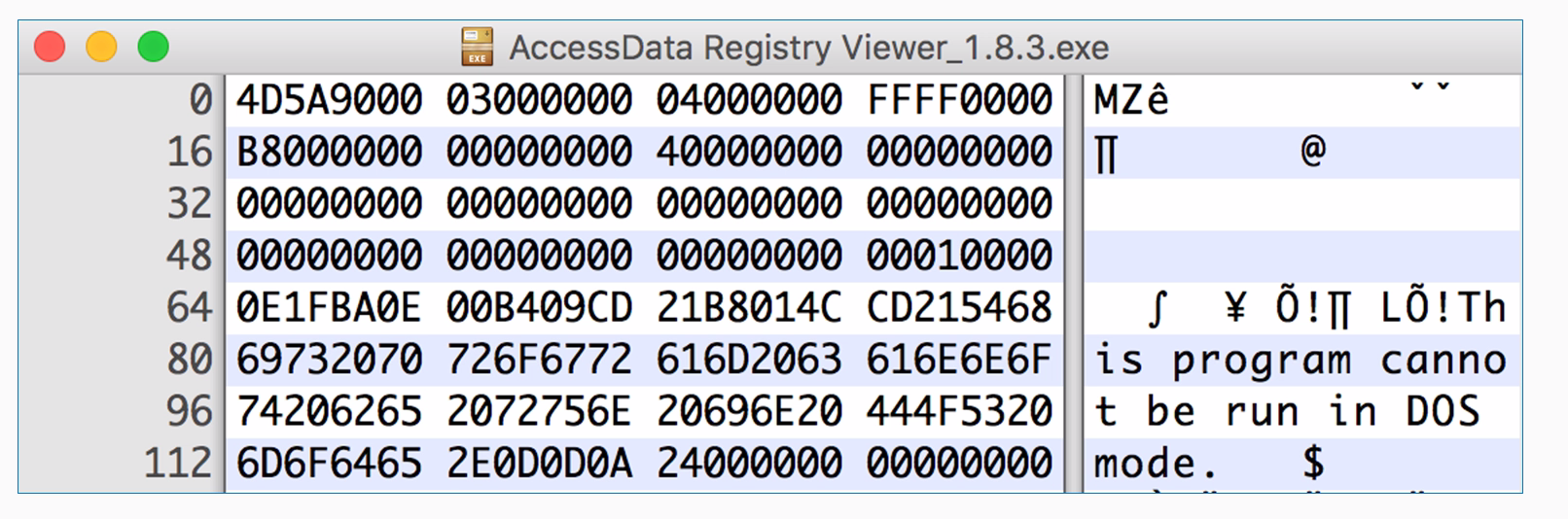As this is a hex viewer, you see the raw data in hexadecimal, and on the right, it attempts to print it as ASCII. Windows programs have this string at the start, and this program cannot be run in DOS mode, but they have a lot of unprintable characters, such as `FF` and `0`, which really doesn't matter for Python at all. An easy way to encode data like that is to read it directly from the file. You can use the`with`command. It will just open a file with filename and mode read binary with the handle`f`and then you can read it. The`with`command is here just to tell Python to open the file, and that if it cannot be opened due to some error, then just to close the handle and then decode it exactly the same way. To decode data you've encoded in this fashion, you just take the output string and you put `.decode` instead of `.encode`.

Now let's take a look at how to handle binary data:

1. We will first exit Python so that we can see the filesystem, and then we'll look for the `Ac` file using the command shown here:
```>>> exit()
\$ ls Ac*
AccessData Registry Viewer_1.8.3.exe```

There's the filename. Since that's kind of a long block, we are just going to copy and paste it.

1. Now we start Python and `clear` the screen using the following command:
`\$ clear`
1. We will start `python` again:
`\$ python`
1. Alright, so, now we use the following command:
```>>> with open("AccessData Registry Viewer_1.8.3.exe", "rb") as f:
... print data.encode("base64")```

Here we enter the filename first and then the mode, which is read binary. We will give it filename handle of `f`. We will take all the data and put it in a single variable data. We could just encode the data in`base64`, and it would automatically print it. If you have an intended block in Python, you have to pressEntertwice so it knows the block is done, and then`base64` encodes it.

1. You get a long block of `base64` that is not very readable, but this is a handy way to handle data like that; say, if you want to email it or put it in some other text format. So, to do the decoding, let's encode something simpler so that we can easily see the result:
```>>> "ABC".encode("base64")
'QUJD\n'```
1. If we want to play with it, put that in a `c`variable using the following command:
```>>> c = "ABC".encode("base64")
>>> print c
QUJD```
1. Now we can print `c` to make sure that we have got what we expected. We have `QUJD`, which is what we expected. So, now we can decode it using the following command:
```>>> c.decode("base64")
'ABC'```

`base64` is not encrypting. It is not hiding anything, but it is just another way to represent it. In the next section, we'll cover XOR.

## XOR

This section explains what XOR is on single bits with a truth table, and then shows how to do it on bytes. XOR undoes itself, so decryption is the same operation as encryption. You can use single bytes or multiple byte keys for XOR, and we will use looping to test keys. Here's the XOR truth table:

• `0 ^ 0 = 0`
• `0 ^ 1 = 1`
• `1 ^ 0 = 1`
• `1 ^ 1 = 0`

If you feed in two bits and the two bits are the same, the answer is `0.` If the bits are different, the answer is `1`.

### Note

XOR operates on one bit at a time. Python indicates XOR with the `^` operator.

The truth table shows how it works. You feed in bits that are equally likely to be `0` and `1` and XOR them together, then you end up with 50% ones and zeros, which means that XOR does not destroy any information.

Here's the XOR for bytes:

• `A 0b01000001`
• `B 0b01000010`
• `XOR 0b00000011`

`A` is the number `65`, so you have `1` for `64` and `1` for `1``B` is `1` larger, and if you XOR the two of them together, all the bits match for the first 6 bits, and they're all `0`. The last two bits are different, and they turn into `1`. This is the binary value `3`, which is not a printable character, but you can express it as an integer.

The key can be single byte or multibyte. If the key is a single byte, such as `B`, then you use the same byte to encrypt every plaintext character. Just keep repeating the key over and over: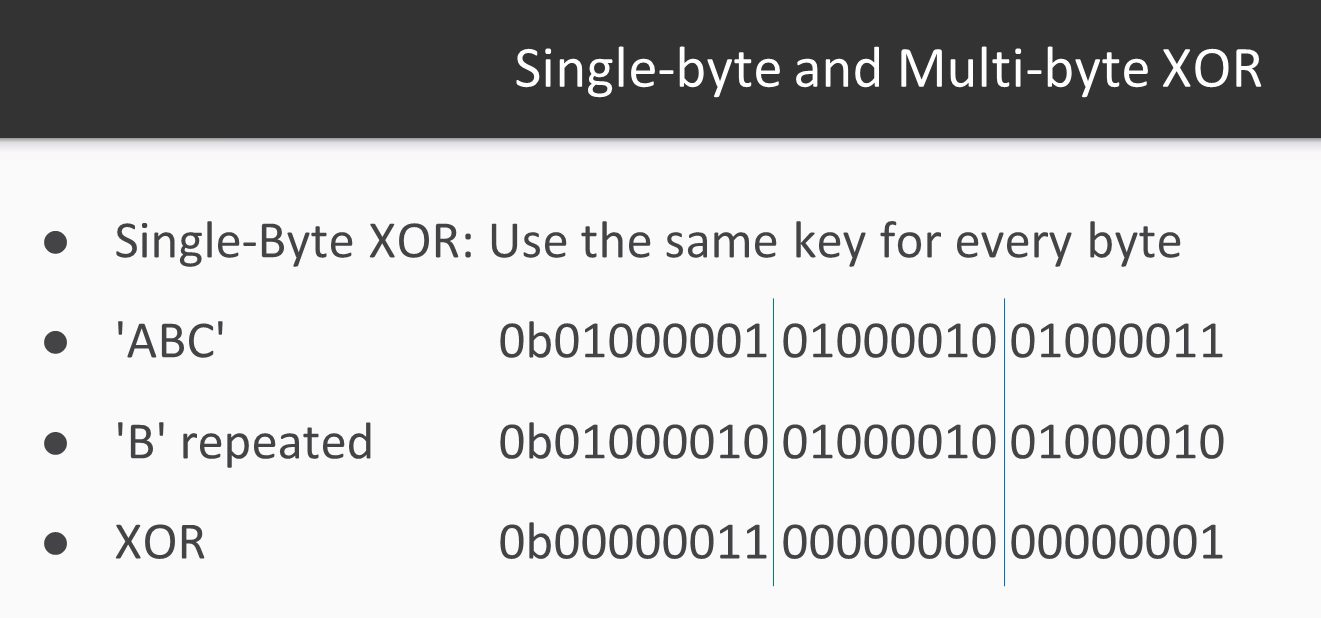Repeat `B` for this byte, `B` for that byte, and so on. If the key is multibyte, then you repeat the pattern: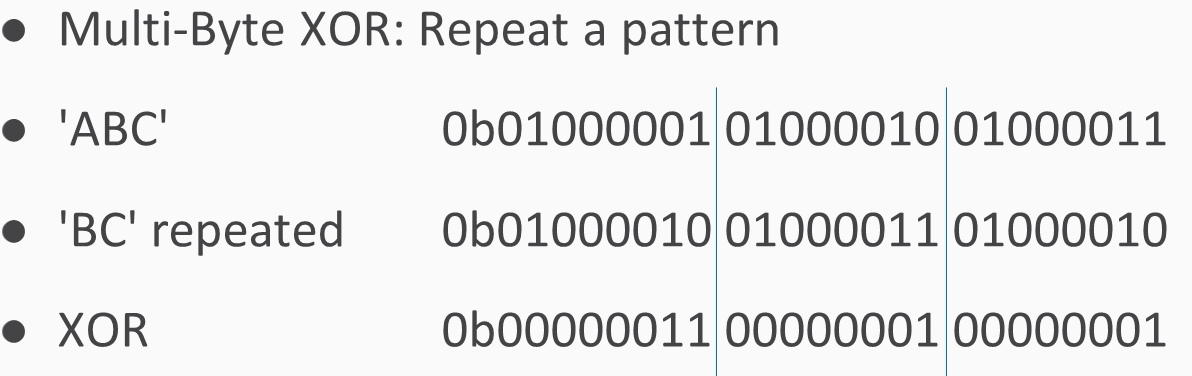You use `B` for the first byte, `C` for the next byte, then again `B` for the next byte, `C` for the next byte, and so on.

To do this in Python, you need to loop through the bytes of a string and calculate an index to show which byte you're on. Then we enter some text from the user, calculate its length, then go through the indices from `1` up to the length of the string, starting at`0`. Then we take the text byte and just print it out here so you can see how the loop works. So, if we give it a five-character plaintext, such as `HELLO`, it just prints out the characters one by one.

To do the XOR, we'll input a plaintext and a key and then take a byte of text and a byte of key, XOR them together, and print out the results

Note `%len( key)`, which is what prevents you from running off the end of the key. It will just keep repeating the bytes in the key. So, if the key is three bytes long, this will be modulus three, so it will count as `0`, `1`, `2`, and then back to `0 1 2 0 1 2`, and so on. In this way, you can handle any length of plaintext.

If you combine uppercase and lowercase letters, you'll often find the case that XOR produces unprintable bytes. In the example that follows, we have used`HELLO``Kitty`, and a key of`qrs`. Note that some of these bytes are readily printable and some of them contain strange characters, such asEsc and Tab, which are difficult to print. Therefore, the best way to handle the output is not to attempt to print it as ASCII, but instead print it as`hex`encoded values. Instead of trying to print the bytes one by one, we combine them into a`cipher`variable, and in the end, we print out the entire plaintext, the entire key, and then the entire ciphertext in hex. In this way, it can correctly handle these strange values that are difficult to print.

Let's try this looping in Python:

1. We open the Terminal and enter the following command:
`\$ nano xor1.py`
1. When you run it, you will get the following output: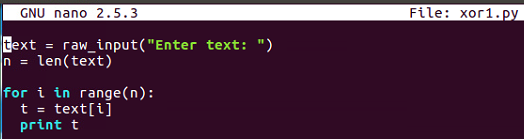1. This is the first one that is `xor1.py`, so we input text from the user, calculate it's length, and then just print out the bytes one by one to see how the loop works. Let's run it and give it `HELLO`: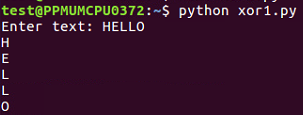1.  It just prints out the bytes one by one. Now, let's look at the next XOR 2: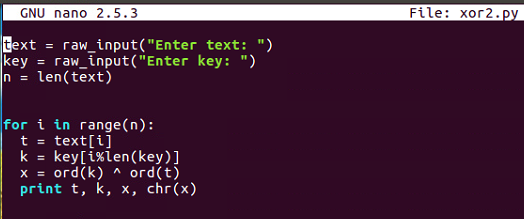This inputs `text` and `key` the same way and goes through each byte of `text`, picks out the correct byte of `key` using the modular arithmetic, performs the XOR, and prints out the results.

1. So if we run the same file here, we take `HELLO` and a `key` as shown:
```\$ nano xor2.py
\$ python xor2.py```

So, the output is as follows: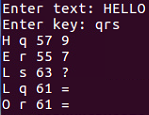It calculates the bytes one by one. Note how we get two equals signs here, which is the reason why you would use a multiple by `key` because the plaintext is changing but the key, is also changing and that pattern is not reflected in the output, so it's more effective obfuscation.

1. Clear that and look at the third `xor2a.py` file: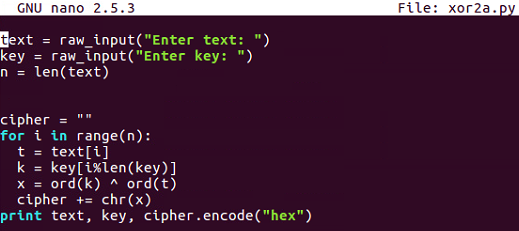You can see that this handles the problem of unprintable bytes.

1. So, we create a variable named `cipher`, combine each byte of output here, and at the end, we encode it with `hex` instead of trying to `print` it out directly: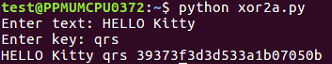1. If you give it `HELLO` and then text a key of `qrs`, it will give you the plaintext `HELLO Kitty`, the key, and then the hexadecimal-encoded output, which can easily handle funny characters, such as `0 7` and `0 5`. In the next section, you'll see challenge 1—the Caesar cipher.

## Challenge 1 – the Caesar cipher

After a Caesar cipher review, we'll have an example of how to solve it and then your challenge. Remember how the Caesar cipher works. You have an alphabet of available characters, you take in the message and a `shift` value, and then you just shift the characters forward that many steps in the alphabet, wrapping around if you go around the end. The script we end up with works for any `shift` value, including normal numbers, such as `3`, or even numbers that are larger than `26`; they just wrap around and can scramble any data you put it.

Here's an example:

1. For ciphertext, you can decipher it by just trying all the `shift` values from `0` to `25`, and one of them will just be readable. This is a simple brute-force attack. Let's take a look at it.

Here, in Python, go to the `caesar4` script, that we had before. It takes in a string and shifts it by any value you specify. If we use that script, we can run it as follows: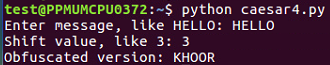1. Then, if we put in `HELLO` and shift it by `3`, it turns into `KHOOR`.
2. If we want to crack it, we can use the solution script as follows: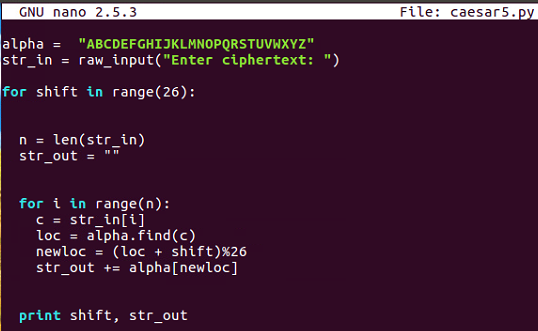1. So, if we use that script, we can run it: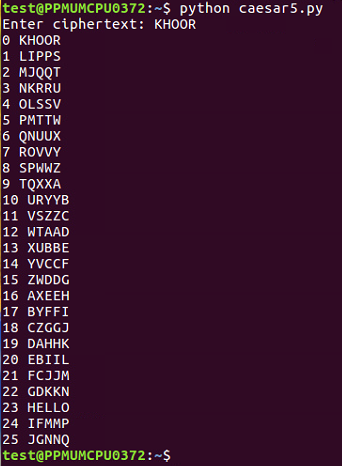1. If we put it in `KHOOR`, it'll shift it by a variety of values, and you can see the one that's readable at `23`, which is `HELLO`. So, the example we discussed before of longer ciphertexts and so on will become readable down at `3`, where you see its `DEMONSTRATION`: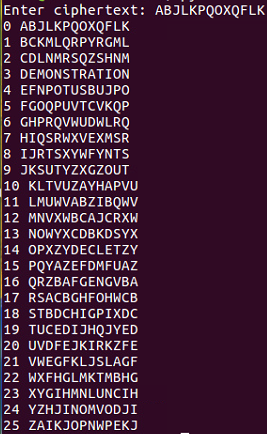1. Your challenge is to decipher this string: `MYXQBKDEVKDSYXC`.

In the next section, we'll have a challenge on `base64`.

## Challenge 2 – base64

After a `base64` review, we'll perform an example to show you how to decode some obfuscated text, and then we have one simple and one hard challenge for you.

Here is the `base64` review: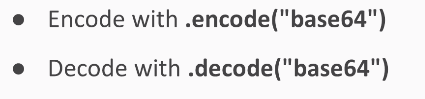`base64` encoding text makes it longer. Here's the sample text to decode:

`U2FtcGxliHRleHQ=`

It decodes into the string sample text. Let's take a look at that.

Refer to the following steps:

1. If you run `python` in immediate mode, it will do four simple jobs:
`\$ python`
1. So, if we take `ABC` and encode it with `base64`, we get this string:
```>>> "ABC".encode("base64")
'QUJD\n'```
1. If we decode that with `base64`, we get back to the original text:
```>>> "QUJD".decode("base64")
'ABC'```
1. So, the challenge text is as follows, and if you decode it, you get the string sample text:
```>>> "U2FtcGxliHRleHQ=".decode("base64")
'Sample text'```
1. So, that will do for simple case; your first challenge looks like that:
`Decode this: VGhpcyBpcyB0b28gZWFzeQ==`
1. Here's a long string to decode for your longer challenge:
```Decode this:
VWtkc2EwbEliSFprVTJeFl6SlZaMWxUUW5OaU1qbDNVSGM5UFFvPQo=```

This long string is so long because it's been encoded by `base64` not just once but several times. So, you'll have to try decoding it until it turns into something readable. In the next section, we'll have Challenge 3 – XOR.

## Challenge 3 – XOR

In this section, we will review how XOR works and then give you an example, and then present you with two challenges.

So, here is one of the XOR programs we discussed before: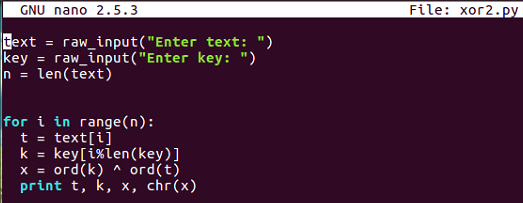You input arbitrary texts and an arbitrary key, and then go through the bytes one by one, picking out one byte of text and one byte of key before combining them with XOR and printing out the results. So, if you put in `HELLO` and `qrs`, you'll get encrypted stuff, encrypted with XOR.

Here's an example: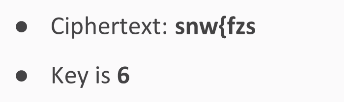It will scramble into `EXAMPLE`. So, this undoes encryption; remember that XOR undoes itself.

If you want to break into one of these, one simple procedure is just to try every key and print out the results for each one, and then read the key is readable.

So, we try all single-digit keys from `0` to `9`.

The result is that you feed in the ciphertext, encrypt it with each of these, and when you hit the correct key value, it will turn into readable text.

Let's take a look at that: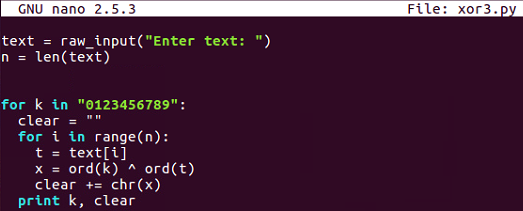Here's the decryption routine, which simply inputs texts from the user and then tries every key in this string, `0` through `9`. For each one of those it combines, think the XORed text into a variable named `clear`, so it can print one line for each key and then the clear result. So, if we run that one and put in my ciphertext, it gives us 10 lines.: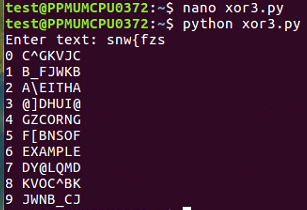We just scanned through these lines and saw which one becomes readable, and you can see the correct key and the correct plaintext at `6`. The first challenge is here: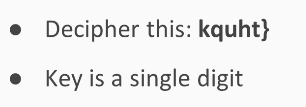This is similar to the one we saw earlier. The key is a single digit, and it will decrypt into something readable. Here's a longer example that is in a hexadecimal format: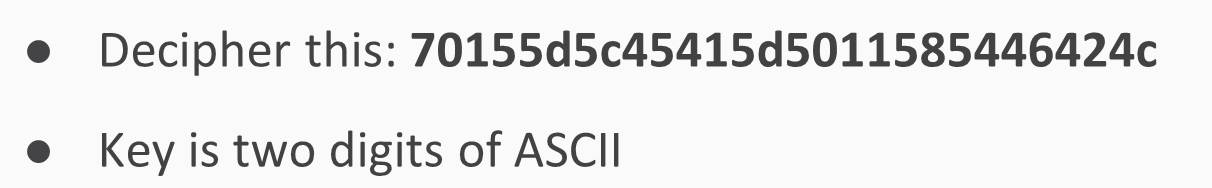The key is two digits of ASCII, so you'll have to try 100 choices to find a way to turn this into a readable string.

## Summary

In this chapter, after setting up Python, we covered the simple substitution cipher, the Caesar cipher, and then `base64` encoding. We gathered data six bits at a time instead of eight bits at a time, and then we looked at XOR encoding, where bits are flipped one by one in accordance with the key. We also saw a very simple truth table. The challenges you performed were cracking the Caesar cipher without the key, cracking `base64` by reversing it to get the original bytes, and cracking XOR encryption without knowledge of the key with a brute-force attack trying all possible keys. In Chapter 2, Hashing, we will cover different types of hashing algorithms.